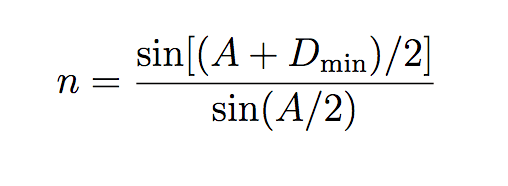# How do I find refractive index uncertainties?

Member advised to use the formatting template for all homework help requests
I've been doing an experiment where I've used prisms and a spectrometer to find the exact angles inside the prisms and the refractive index of the prisms by finding the minimum angle of deviation.

I have attached a picture of the formula I've been using to find the refractive indices. Where Dmin in the minimum angle of deviation and A is the measurement I've made of the angles in the prism (both of which in themselves have uncertainties).I'm now trying to find the uncertainty in my value for the refractive index but I'm really struggling because there are so many components to go through. I'd be really grateful if someone could talk me through the easiest way of finding the uncertainty in my refractive index values.

DrDu